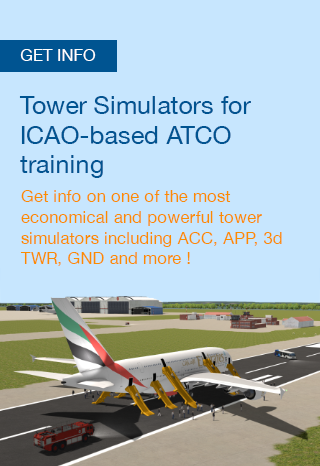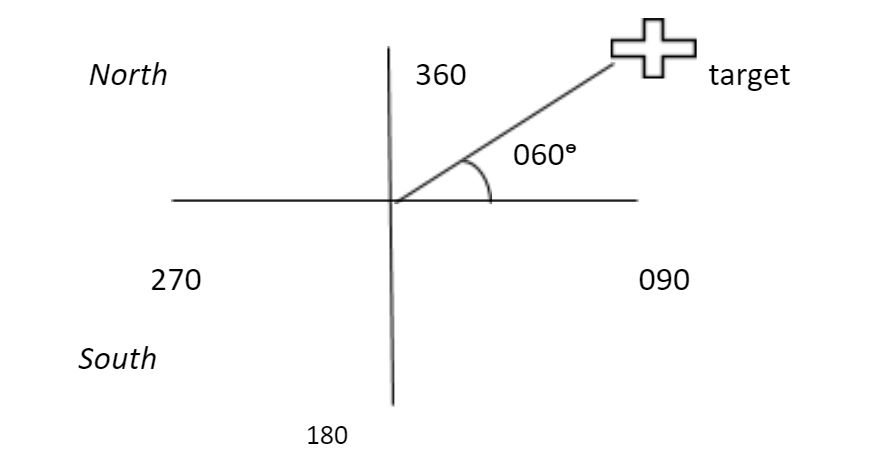RADAR has become a key technology to help navigate aircraft. With a growing demand of efficient air space usage, the demand for RADAR systems is growing. RADAR is a technology that uses Radio waves to detect objects in the air or sea.

Range and Bearing are one of two main characteristics that are measured by the RADAR. In order to understand these two parameters we need to understand how a RADAR works.

##hbspt.cta._relativeUrls=true;hbspt.cta.load(2968615, '4c52de5c-81ad-4380-8b30-67794153a89b', {"useNewLoader":"true","region":"na1"}); RADAR

Radar is an acronym for "radio detection and ranging." A radar system usually operates in the ultra-high-frequency (UHF) or microwave part of the radio-frequency (RF) spectrum, and is used to detect the position and/or movement of objects.

## Range

Range in simpler words is the distance of an object from a particular point. In radar, range is derived through a formula.  But why do you need a formula to get the range acquired through a RADAR?

Because RADAR technology uses Radio waves that travel at the speed of light.  As mentioned range is a distance of target from a particular object. To measure distance we use Newton's formula

S=v*t

Let us measure distance of an object in this case an aircraft.  The distance of an object from the RADAR is called slant range - it is along the line of sight.

Slant range is the line of sight distance between the radar and the object illuminated. The ground range is the horizontal distance between the emitter and its target. Its calculation requires knowledge of the target's elevation.

Since the radio waves are traveling at the speed of light let us call this speed Co

The formula will be

V=s/t

Co =2R/t

Whereas “2R” is the observation of the radar pulse traveling to the target and then coming back to the radar.  “t” is the time taken.

Now V= Co for the velocity of light which is C= 3·108 m/s

Distance S=2R.

T is the time taken.

The formula derived for the range consequently is: R= Co * t/2

The range formula is calculated by RADAR software module.

## Bearing

In navigation “Bearing” describes the horizontal angle between the direction of an object and another object or between it and true north. It is measured in mils or degrees. Bearing is used in civil aviation, warfare as well as search and rescue.

In RADAR technology bearing is the determination of the direction. The True Bearing (referenced to true north) of a radar target is the angle between the true north and a line pointed directly at the target. This angle is measured in the horizontal plane and in a clockwise direction from true north.

The bearing angle to the radar target may also be measured in a clockwise direction from the centerline of your own ship or aircraft. It is referred to as the relative bearing.

In aircraft navigation, an angle is normally measured from the aircraft's track or heading in a clockwise direction.

Modern radar sets take on this task and with the help of the GPS satellites determine the north direction independently.RADAR has become a key technology to help navigate aircraft. With a growing demand of efficient air space usage, the demand for RADAR systems is growing. RADAR is a technology that uses Radio waves to detect objects in the air or sea.

Range and Bearing are one of two main characteristics that are measured by the RADAR. In order to understand these two parameters we need to understand how a RADAR works.

##hbspt.cta._relativeUrls=true;hbspt.cta.load(2968615, '4c52de5c-81ad-4380-8b30-67794153a89b', {"useNewLoader":"true","region":"na1"}); RADAR

Radar is an acronym for "radio detection and ranging." A radar system usually operates in the ultra-high-frequency (UHF) or microwave part of the radio-frequency (RF) spectrum, and is used to detect the position and/or movement of objects.

## Range

Range in simpler words is the distance of an object from a particular point. In radar, range is derived through a formula.  But why do you need a formula to get the range acquired through a RADAR?

Because RADAR technology uses Radio waves that travel at the speed of light.  As mentioned range is a distance of target from a particular object. To measure distance we use Newton's formula

S=v*t

Let us measure distance of an object in this case an aircraft.  The distance of an object from the RADAR is called slant range - it is along the line of sight.

Slant range is the line of sight distance between the radar and the object illuminated. The ground range is the horizontal distance between the emitter and its target. Its calculation requires knowledge of the target's elevation.

Since the radio waves are traveling at the speed of light let us call this speed Co

The formula will be

V=s/t

Co =2R/t

Whereas “2R” is the observation of the radar pulse traveling to the target and then coming back to the radar.  “t” is the time taken.

Now V= Co for the velocity of light which is C= 3·108 m/s

Distance S=2R.

T is the time taken.

The formula derived for the range consequently is: R= Co * t/2

The range formula is calculated by RADAR software module.

## Bearing

In navigation “Bearing” describes the horizontal angle between the direction of an object and another object or between it and true north. It is measured in mils or degrees. Bearing is used in civil aviation, warfare as well as search and rescue.

In RADAR technology bearing is the determination of the direction. The True Bearing (referenced to true north) of a radar target is the angle between the true north and a line pointed directly at the target. This angle is measured in the horizontal plane and in a clockwise direction from true north.

The bearing angle to the radar target may also be measured in a clockwise direction from the centerline of your own ship or aircraft. It is referred to as the relative bearing.

In aircraft navigation, an angle is normally measured from the aircraft's track or heading in a clockwise direction.

Modern radar sets take on this task and with the help of the GPS satellites determine the north direction independently.